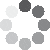Enter parameters Length & Width
Enter parameters
Length:
ft
• inches (in)
• feet (ft)
• yards (yd)
• centimeters (cm)
• meters (m)
Width:
ft
• inches (in)
• feet (ft)
• yards (yd)
• centimeters (cm)
• meters (m)
Plants Spacing:
in
• inches (in)
• feet (ft)
• yards (yd)
• centimeters (cm)
• meters (m)
Planting Pattern:
Square
Triangle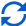Result
Area:
0
ft²
• square feet (ft²)
• square inches (in²)
• square yards (yd²)
• square miles (mi²)
• acres (ac)
• square centimeters (cm²)
• square meters ()
NUMBER OF PLANTS:
0
Enter parameters
Area:
ft²
• square feet (ft²)
• square inches (in²)
• square yards (yd²)
• square miles (mi²)
• acres (ac)
• square centimeters (cm²)
• square meters ()
Plants Spacing:
in
• inches (in)
• feet (ft)
• yards (yd)
• centimeters (cm)
• meters (m)
Planting Pattern:
Square
TriangleResult
NUMBER OF PLANTS:
0(No Ratings Yet)Loading...
Similar Calculators:
Real-time graphics. Make the calculations and see the changes.
Real-time graphics. Make the calculations and see the changes.
Embed
Similar Calculators:Trying to figure out how many bushes/shrubs/trees/groundcover plants you need for your garden?

We know how tricky working this out can be!

Using our plant spacing calculator, figuring this out will take a matter of seconds, saving you time and money on your next landscape project.

Based on the measurements you provide, it can calculate the area of garden you need to cover and how many plants you will need.

Contents:

## Plant Calculator Formula

The plant quantity calculator works out the area of the garden based on the measurements you provide (in metric or imperial units) using the formula:

$$Area\,of\,garden = Length \times Width$$

Our calculator allows you to use both square and triangular patterns for the plants with equal coverage.

All you need to do is decide on the distance between each plant.

### Square Spacing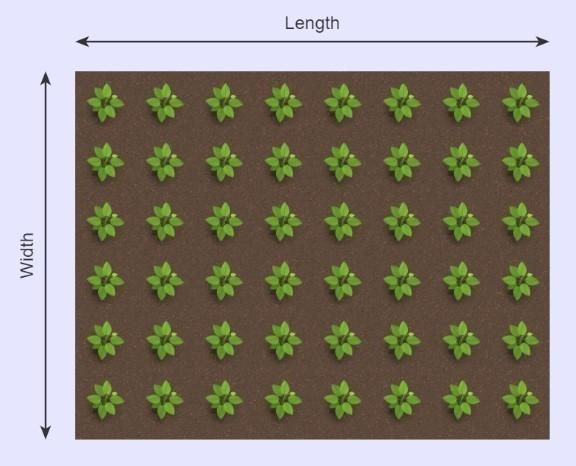To work out how many plants your landscape project needs, the calculator works out how many plants can fit in each row and each column:

$$Plants\,per\,row = {Length \over Plant\,spacing}$$

$$Plants\,per\,column = {Width \over Plant\,spacing}$$

The calculator works out the total quantity of plants your garden needs by multiplying these values together:

$$Total\,number\,of\,plants = Plants\,per\,row × Plants\,per\,column$$

Warning!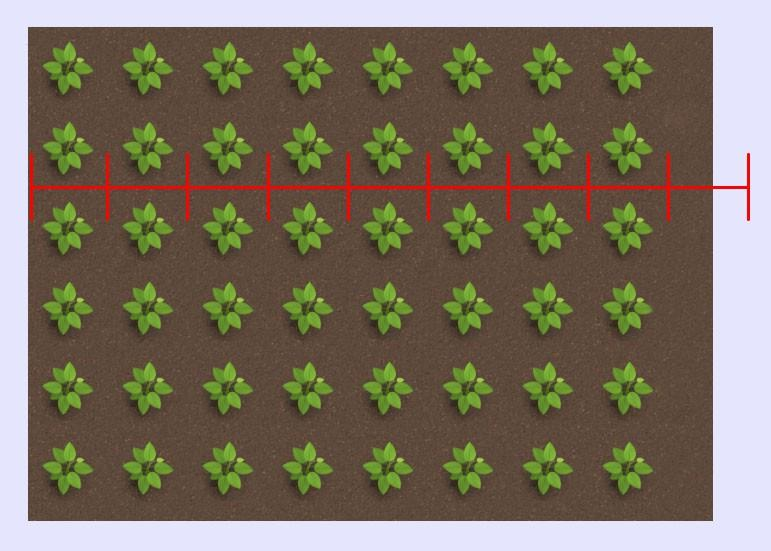Many online calculators use this formula:

$$Total\,number\,of\,plants = {Area\,of\,garden \over Plant\,spacing^2}$$

This formula is incorrect because it fails to account for the fact that plants must be placed in rows and columns.

It assumes that any left-over space can be used for extra plants, ruining your pattern.

As you can see in the picture, there isn’t enough space for another column of plants, but the above formula would not take this into account!

Our calculator does.

### Triangular Spacing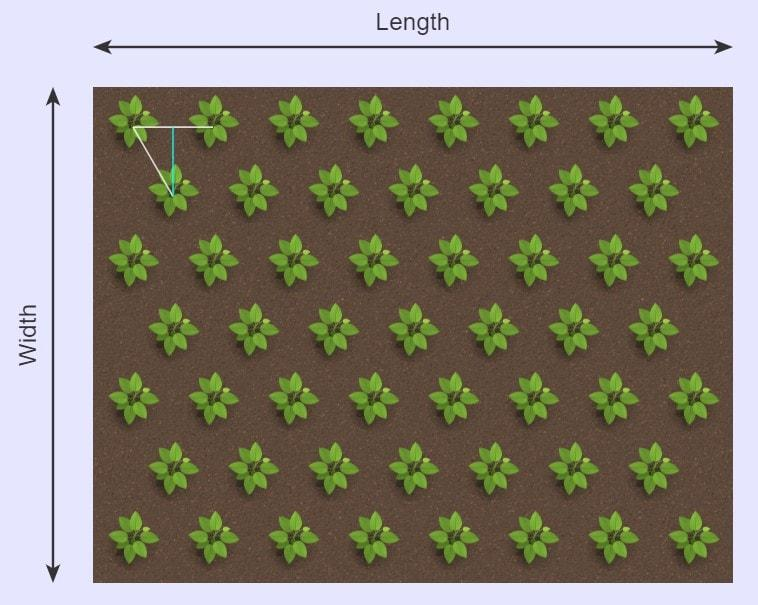If you want triangular spacing, then you need to place the plants on the corners of equilateral triangles (equal distance apart at 60 degrees).

Our calculator can work out the vertical row spacing (the blue line) given you know the horizontal and diagonal spacing (the plant spacing!):

$$Row\,spacing = Plant\,spacing \times 0.866$$

In rows 1, 3, 5, etc., (the odd numbers!):

$$Plant\,Number\,(O) = {Length \over Plant\,spacing}$$

Now, in row 2, 4, 6, etc., (the event numbers):

$$Plant\,Number\,(E) = {Length-Plant\,spacing × 0.5 \over Plant\,spacing}$$

This accounts for the plants in the second row being moved right by half a space compared to the first row.

Next, to calculate the total number of rows:

$$Total\,rows = {Width-Plant\,spacing \over Row\,spacing} + 1$$

The number of even rows (2, 4, 6, etc.) can be given by:

$$Rows\,(E) = {Total\,number\,of\,rows \over 2}$$

Therefore, the number of odd rows (1, 3, 5, etc.) is given by:

$$Rows\,(O) = Total\,rows – Rows\,(E)$$

Finally, the calculator works out the total count for the number of plants you will require:

$$Total\,number\,of\,plants = Plant\,number\,(E) \times Rows\,(E) + Plant\,number\,(O) \times Rows\,(O)$$

Warning

Like all planting calculators, you need to use whole numbers for the formulae.

All measurements for the number of rows, plants, etc., must also be rounded down (you can’t have half a tree!)

Confused?

See below for two full examples.

## Example calculations

• Length and Area with Square Planting Pattern

Let’s assume I have a ground space measuring 100 feet by 50 feet and I want to plant trees with 6-foot spacing in a square pattern.

I enter these values into the calculator as shown:

$$Area = Length \times Width = 100\,ft \times 50\,ft = 5000\,ft^2$$

$$Plants\,Per\,Row = {Length \over Plant\,Spacing} = {100\,ft \over 6\,ft} = 16$$

$$Plants\,Per\,Column = {Width \over Plant\,Spacing} = {50\,ft \over 6\,ft} = 8$$

Note how these values are rounded down.

Therefore, I need to purchase  trees to cover the 5000 square footage of land.

• Length and Area with a Triangular Planting Pattern

Let’s now imagine I want to plant the same ground space (100 ft × 50 ft) with flowers in a triangular pattern.

I enter the values in the calculator as shown:

$$Row\,spacing = Plant\,spacing \times 0.866 = 6 \times 0.866 = 5.196\,ft$$

$$Plant\,Number\,(O) = {Length \over Plant\,spacing} = {100 \over 6} = 16$$

$$Plant\,Number\,(E) = {Length – Plant\,spacing \times 0.5 \over Plant\,spacing} = {100 – 6 \times 0.5 \over 6} = 16$$

$$Total\,rows= {Width-Plant\,spacing \over Row\,spacing} + 1 = {50-6 \over 5.196} + 1 = 9$$

$$Rows\,(E)= {Total\,number\,of\,rows \over 2} = {9 \over 2} = 4.5 \rightarrow 4$$

$$Rows\,(O) = Total\,rows – Rows\,(E) = 9 – 4 = 5$$

Again, note how these values are all rounded down.

There are 5 odd rows (rows 1,3,5,7,9) with 16 flowers per row, and 4 even rows (rows 2,4,6,8) again with 16 flowers each.

Therefore,

$$Total\,number\,of\,plants = Plant\,number\,(E) \times Rows\,(E) + Plant\,number\,(O) \times Rows\,(O) = 4 \times 16 + 5 \times 16 = 144$$

Embed this calculator on your site!Add live graphics
Copied to clipboard! Preview